# JEE Main 2020 Physics (Shift 2-2nd Sept) Paper With Solutions

JEE Main 2020 Physics 2nd September shift 2 solved questions are available here. The subject experts at BYJU’S have solved all these questions. Students can also download these solutions from our website in PDF format. Good understanding of the core concepts of the subjects, and practising the application of those concepts by solving previous years’ question papers will help the students to score well in the competitive exam. Practising sample question paper helps the candidate to inculcate important skills such as attempting each problem with the best possible approach for the fastest solution.

### September 2 Shift 2 - Physics

1. If momentum (P), area (A) and time (T) are taken to be the fundamental quantities then the dimensional formula for energy is:

1. 1) [P1/2 AT–1]
2. 2) [PA1/2 T–1]
3. 3) [PA ½ T–1]
4. 4) [P2 AT–2]

Solution:

$$\left [ P \right ]=MLT^{-1}\leftarrow momentum$$

$$\left [ A \right ]=M^{0}L^{2}T^{0}\leftarrow Area$$

$$\left [ T \right ]=M^{0}L^{0}T^{1}\leftarrow Time$$

$$\left [ E \right ]=P^{x}A^{y}T^{z}$$

$$ML^{2}T^{-2}=\left [ MLT^{-1} \right ]^{x}\left [ L^{2} \right ]^{y}\left [ T \right ]^{z}$$

=MxLx+2y Tz-x

Comparing both sides :-

x=1…………………………(1)

x+2y=2⇒ 1+ 2y = 2 or y= (1/2)……….(2)

z-x = -2 ⇒ z-1 = -2 or z=-1………………(3)

Therefore,

$$\left [ E \right ]=\left [ P^{1}A^{1/2}T^{-1} \right ]$$

2. Two uniform circular discs are rotating independently in the same direction around their common axis passing through their centres. The moment of inertia and angular velocity of the first disc are 0.1 kg –m2 and 10 rad s–1 respectively while those for the second one are 0.2 kg–m2 and 5 rad s–1 respectively. At some instant they get stuck together and start rotating as a single system about their common axis with some angular speed. The Kinetic energy of the combined system is:

1. 1) 2/3 J
2. 2) 10/3 J
3. 3) 5/3 J
4. 4) 20/3 J

Solution: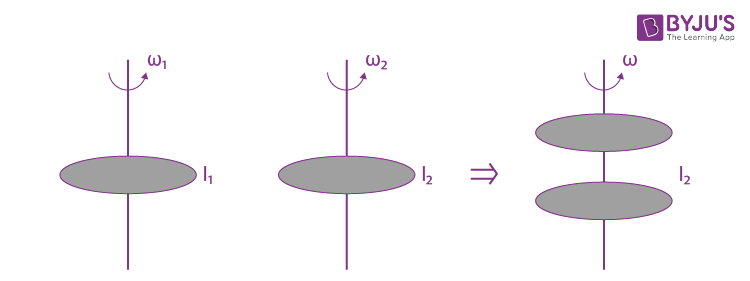$$I_{1}\omega _{1}+I_{2}\omega _{2}=(I_{1}+I_{2})\omega$$

$$\omega =\frac{I_{1}\omega _{1}+I_{1}\omega _{2}}{I_{1}+I_{2}}=\frac{0.1\times 10+0.2\times 5}{0.1+0.2}=\frac{1+1}{0.3}=\frac{2}{0.3}$$

$$\omega =\frac{20}{3}$$

Now find KE =

$$\frac{1}{2}I_{1}\omega ^{2}+\frac{1}{2}I_{2}{\omega ^{2}}$$

$$\frac{1}{2}(I_{1}+I_{2})\omega ^{2}$$

$$\frac{1}{2}\times 0.3\times \left ( \frac{20}{3} \right ) ^{2}$$

(KE)f =(20/3)

3. A particle is moving 5 times as fast as an electron. The ratio of the de-Broglie wavelength of the particle to that of the electron is 1.878 × 10–4. The mass of the particle is close to:

1. 1) 4.8 × 10–27 kg
2. 2) 9.1 × 10–31 kg
3. 3) 9.7 × 10–28 kg
4. 4) 1.2 × 10–28 kg

Solution:

$$p = \frac{h}{\lambda }$$

$$\lambda = \frac{h}{P }$$

$$\frac{\lambda _{particle}}{\lambda _{e}}=1.878 \times 10^{-4}$$

$$\Rightarrow \frac{h}{P_{paticle}}\times \frac{Pe}{h}=1.878 \times 10^{-4}$$

$$\Rightarrow \frac{M_{e}V_{e}}{M_{p}V_{p}}=1.878 \times 10^{-4}$$

$$\Rightarrow M_{p}=\frac{M_{e}}{1.878\times 10^{-4}}\times \left ( \frac{V_{e}}{V_{p}} \right )$$

$$=\frac{9.11\times 10^{-31}}{1.878\times 10^{-4}}\times \left ( \frac{1}{5} \right )$$

= 9.7 x 10-28 Kg

4. A potentiometer wire PQ of 1 m length is connected to a standard cell E1. Another cell E2 of emf 1.02 V is connected with a resistance ‘r’ and switch S (as shown in figure). With switch S open, the null positon is obtained at a distance of 49 cm from Q. The potential gradient in the potentiometer wire is:1. 1) 0.03V/cm
2. 2) 0.02 V/cm
3. 3) 0.04 V/cm
4. 4) 0.01 V/cm

Solution: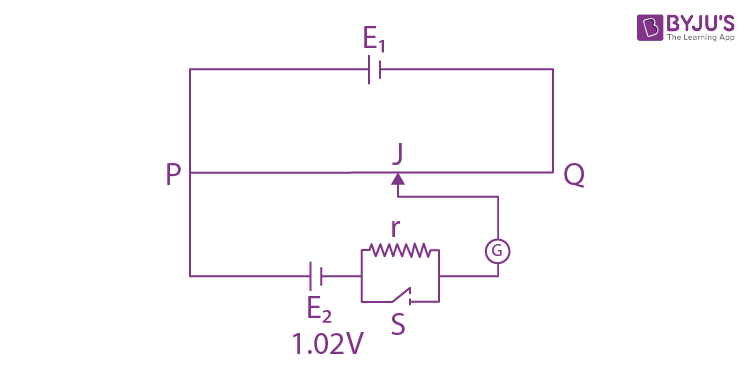PQ= 1 m

QJ = 49 cm

PJ = 51 cm

V/l = 1.02/51 = 0.02 v/cm

5. In the following digital circuit, what will be the output at ‘Z’, when the input (A,B) are (1,0), (0,0), (1,1), (0,1):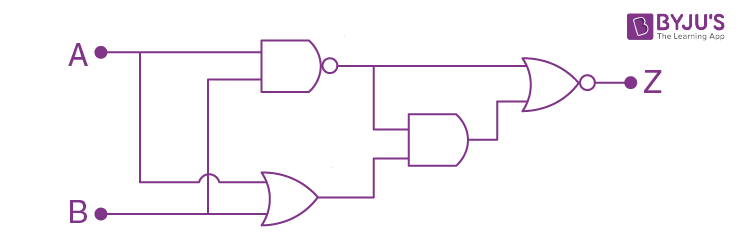1. 1) 0,1,0,0
2. 2) 1,1,0,1
3. 3) 0,0,1,0
4. 4) 1,0,1,1

Solution: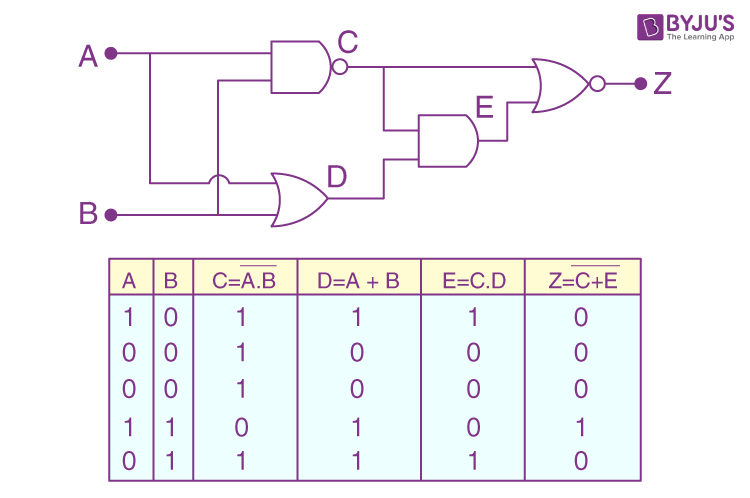6. A wire carrying current I is bent in the shape ABCDEFA as shown, where rectangle ABCDA and ADEFA are perpendicular to each other. If the sides of the rectangles are of lengths a and b, then the magnitude and direction of magnetic moment of the loop ABCDEFA is:1. 1)
$$\sqrt{2}abI,along \left [ \frac{\hat{j}}{\sqrt{5}}+\frac{2\hat{k}}{\sqrt{5}} \right]$$
2. 2)
$$abI,along \left [ \frac{\hat{j}}{\sqrt{5}}+\frac{2\hat{k}}{\sqrt{5}} \right ]$$
3. 3)
$$\sqrt{2}abI,along \left [ \frac{\hat{j}}{\sqrt{2}}+\frac{\hat{k}}{\sqrt{2}} \right ]$$
4. 4)
$$abI,along \left [ \frac{\hat{j}}{\sqrt{2}}+\frac{\hat{k}}{\sqrt{2}} \right ]$$

Solution:

Loop ABCD

$$\vec{M_{1}}=(abI)\hat{k}$$

Loop DEFA

$$\vec{M_{2}}=(abI)\hat{j}$$

$$\vec{M}=\vec{M_{1}}+\vec{M_{2}}=abI(\hat{j}+\hat{k})$$

$$\left | \vec{M} \right |=\sqrt{2}abI$$

Direction=

$$along \left [ \frac{\hat{j}}{\sqrt{2}}+\frac{\hat{k}}{\sqrt{2}} \right ]$$

$$\sqrt{2}abI,along \left [ \frac{\hat{j}}{\sqrt{2}}+\frac{\hat{k}}{\sqrt{2}} \right ]$$

7. A small point mass carrying some positive charge on it, is released from the edge of a table. There is a uniform electric field in this region in the horizontal direction. Which of the following options then correctly describe the trajectory of the mass? (Curves are drawn schematically and are not to scale).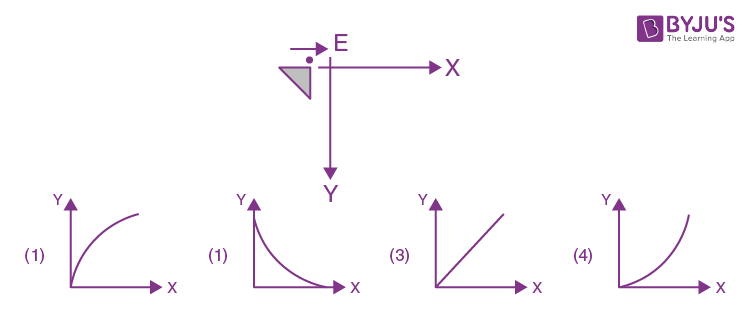Solution: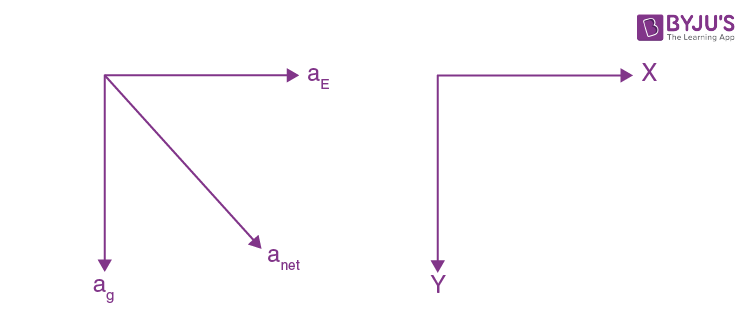Since it is released from rest.

And anet is constant.

If will have straight line path along net ‘a’.

8. In a plane electromagnetic wave, the directions of electric field and magnetic field are represented by

$$\hat{k}$$
and
$$2\hat{i}-2\hat{j}$$
, respectively. What is the unit vector along direction of propagation of the wave.

1. 1)
$$\frac{1}{\sqrt{2}}(\hat{i}+\hat{j})$$
2. 2)
$$\frac{1}{\sqrt{5}}(2\hat{i}+\hat{j})$$
3. 3)
$$\frac{1}{\sqrt{5}}(\hat{i}+2\hat{j})$$
4. 4)
$$\frac{1}{\sqrt{2}}(\hat{j}+\hat{k})$$

Solution:

$$\vec{E}\times\vec{B} =\hat{k}\times (2\hat{i}-2\hat{j})=2\hat{k}\times \hat{i}-2\hat{k}\times \hat{j} = 2\hat{j}+2\hat{i}$$

Unit vector along

$$\vec{E}\times\vec{B} = \frac{1}{2\sqrt{2}}(2\hat{i}+2\hat{j})=\frac{1}{\sqrt{2}}(\hat{i}+\hat{j})$$

C =

$$\frac{1}{\sqrt{2}}(\hat{i}+\hat{j})$$

9. An inductance coil has a reactance of 100 Ω. When an AC signal of frequency 1000 Hz is applied to the coil, the applied voltage leads the current by 45°. The self-inductance of the coil is:

1. 1) 6.7 × 10–7 H
2. 2) 5.5 × 10–5 H
3. 3) 1.1 × 10–1 H
4. 4) 1.1 × 10–2 H

Solution:

L-R circuit

Tan450 = XL/R ⇒ XL= R

Now

$$Z=\sqrt{R^{2}+X_{L}^{2}}$$

Or

$$Z=\sqrt{X_{L}^{2}+X_{L}^{2}}$$

$$=\sqrt{2X_{L}^{2}}=\sqrt{2}X_{L}$$

$$100=\sqrt{2}X_{L}$$

$$X_{L}=\frac{100}{\sqrt{2}}$$

$$\omega _{L}=\frac{100}{\sqrt{2}}\Rightarrow L=\frac{100}{\sqrt{2}\omega }=\frac{100}{\sqrt{2}\times 2\pi f}=\frac{100}{\sqrt{2}\times 2\times 3.14\times 1000}$$

= 1.1 x 10-2 H

10. This displacement time graph of a particle executing S.H.M. is given in figure : (sketch is schematic and not to scale)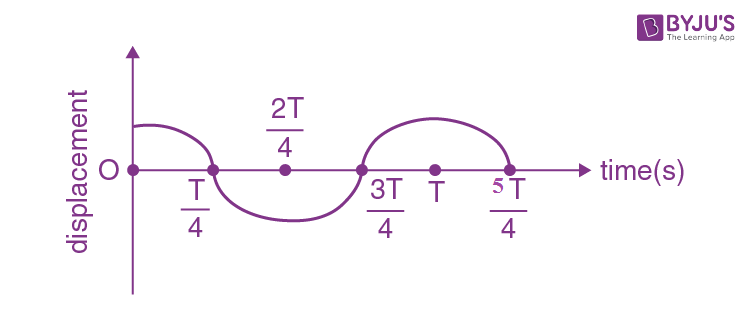Which of the following statements is/are true for this motion?

(A) The force is zero at t = 3T/4

(B) The acceleration is maximum at t=T

(C) The speed is maximum at t = T/4

(D) The P.E. is equal to K.E. of the oscillation at t = T/2

1. 1) (B), (C) and (D)
2. 2) (A), (B) and (D)
3. 3) (A) and (D)
4. 4) (A), (B) and (C)

Solution:

(A) at t= 3T/4

Particle is at mean position

a = 0

F = 0

(B) at t= T,

Particle is at extreme.

F is maximum

a = max

(C) at t =T/ 4 ; mean position

so, maximum velocity

(d) KE = PE

$$\frac{1}{2}k(A^{2}-x^{2})=\frac{1}{2}kx^{2}$$

$$(A^{2}-x^{2})=x^{2}$$

$$A^{2}=2x^{2}$$

$$A=\sqrt{2}x$$

$$x=\frac{A}{\sqrt{2}}=Acos\omega t$$

$$cos\omega t=\frac{1}{\sqrt{2}}$$

$$\omega t=\frac{\pi }{4}$$

$$\frac{2\pi }{T}.t=\frac{\pi }{4}\Rightarrow t=\frac{T}{8}$$

11. In a Young’s double slit experiment, 16 fringes are observed in a certain segment of the screen when light of wavelength 700 nm is used. If the wavelength of light is changed to 400 nm, the number of fringes observed in the same segment of the screen would be:

1. 1) 28
2. 2) 24
3. 3) 30
4. 4) 18

Solution:

$$y =\frac{D\lambda }{d}$$

$$n_{1}\frac{D\lambda_{1} }{d}=n_{2}\frac{D\lambda_{2} }{d}$$

$$\frac{n_{1}}{n_{2}}=\frac{\lambda _{2}}{\lambda _{1}}$$

$$n_{2}=n_{1}\frac{\lambda _{2}}{\lambda _{1}}\Rightarrow 16\times \frac{700}{400}=28$$

12. A heat engine is involved with exchange of heat of 1915 J, –40J, + 125J and –QJ, during one cycle achieving an efficiency of 50.0%. The value of Q is:

1. 1) 980 J
2. 2) 640 J
3. 3) 40 J
4. 4) 400 J

Solution:

$$\eta =\frac{W}{\sum Q_{+}}=\frac{Q_{1}+Q_{2}+Q_{3}+Q_{4}}{Q_{1}+Q_{3}}$$

$$0.5 =\frac{1915-40+125-Q}{1915+125}$$

1020= 1915 -40+125 –Q

Q= 980 J

13. In a hydrogen atom the electron makes a transition from (n + 1)th level to the nth level. If n>>1, the frequency of radiation emitted is proportional to:

1. 1) 1/n2
2. 2) 1/n
3. 3) 1/n3
4. 4) 1/n4

Solution:

$$E_{n}=\frac{-Rhc}{n^{2}}$$

$$E_{n+1}=\frac{-Rhc}{(n+1)^{2}}$$

$$\Delta E=E_{n+1}-E_{n}$$

$$v=Rc\left [ \frac{(n+1)^{2}-n^{2}}{n^{2}(n+1)^{2}} \right ]$$

$$v=Rc\left [ \frac{1+2n}{n^{2}(n+1)^{2}} \right ]$$

If n≥1

$$v=\frac{2n}{n^{2}\times n^{2}}=\frac{2n}{n^{4}}=\frac{2}{n^{3}}$$

v ∝(1/n3)

14. When the temperature of a metal wire is increased from 0°C to 10°C, its length increases by 0.02%. The percentage change in its mass density will be closest to:

1. 1) 0.06
2. 2) 0.008
3. 3) 2.3
4. 4) 0.8

Solution:

∆l / l = ∝∆t

$$\alpha =\frac{\Delta l}{l\Delta t}=\frac{0.02}{100\times 10}=2\times 10^{-5}$$

$$\gamma =3\alpha =6\times 10^{-5}$$

ℓ = m / v

∆ ℓ / ℓ = ∆m / m - ∆v / v

Here, ∆ m / m = 0

∆ ℓ / ℓ = - ∆v / v

Now,

$$\frac{\Delta v}{v}\times 100=\gamma \Delta t.100=6\times 10^{-5}\times 10\times 100$$

=0.06

15. A charge Q is distributed over two concentric conducting thin spherical shells radii r and R (R > r). If the surface charge densities on the two shells are equal, the electric potential at the common centre is:1. 1)
$$\frac{1}{4\pi \epsilon _{0}}\frac{(2R+r)}{R^{2}+r^{2}}Q$$
2. 2)
$$\frac{1}{4\pi \epsilon _{0}}\frac{(R+r)}{R^{2}+r^{2}}Q$$
3. 3)
$$\frac{1}{4\pi \epsilon _{0}}\frac{(R+r)}{2(R^{2}+r^{2})}Q$$
4. 4)
$$\frac{1}{4\pi \epsilon _{0}}\frac{(R+2r)}{2(R^{2}+r^{2})}Q$$

Solution: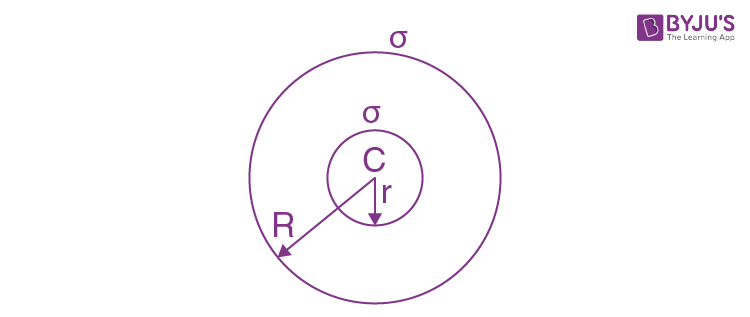Q1= σ4πr2

Q2= σ4πR2

Q=σ4π(R2+r2)

$$\Rightarrow \sigma =\frac{Q}{4\pi (R^{2}+r^{2})}$$

$$V_{c} =\frac{kQ_{1}}{r}+\frac{kQ_{2}}{R}$$

$$=\frac{k\sigma 4\pi r^{2}}{r}+\frac{k\sigma 4\pi R^{2}}{R}=k\sigma 4\pi (r+R)$$

$$=4\pi K\frac{Q}{4\pi (R^{2}+r^{2})}(r+R)=\frac{KQ(r+R)}{R^{2}+r^{2}}$$

$$=\frac{1}{4\pi \epsilon _{0}}\frac{Q(r+R)}{R^{2}+r^{2}}$$

16. A 10 μF capacitor is fully charged to a potential difference of 50V. After removing the source voltage it is connected to an uncharged capacitor in parallel. Now the potential difference across them becomes 20 V. The capacitance of the second capacitor is:

1. 1) 15 μF
2. 2) 20 μF
3. 3) 10 μF
4. 4) 30 μF

Solution: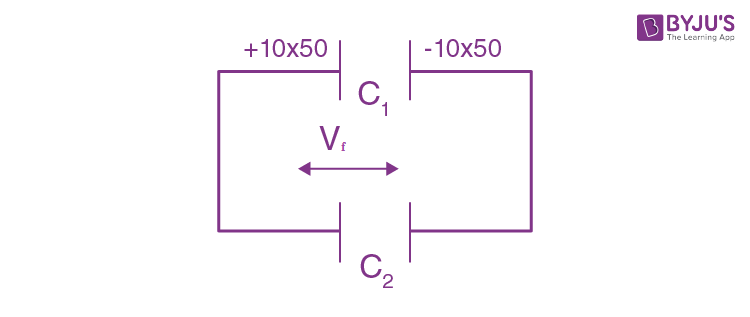C1 = 10 μF

Vf =20V

500/(C1+C2) =20

500/(10+C2) =20

(10+C2) =(500/20) =25

C2= 15 μF

17. An ideal gas in a closed container is slowly heated. As its temperature increases, which of the following statements are true?

(A) the mean free path of the molecules decreases.

(B) the mean collision time between the molecules decreases.

(C) the mean free path remains unchanged.

(D) the mean collision time remains unchanged.

1. 1) (B) and (C)
2. 2) (A) and (B)
3. 3) (C) and (D)
4. 4) (A) and (D)

Solution:

$$\lambda =\frac{1}{\sqrt{2}(\frac{N}{V})\pi d^{2}}$$

λ=Mean free path

N = No. 7 molecules

v = volume of container

d = diameter of molecule

Since,N and V are constant

Since,Mean free path reamains unchanged.

Now, If T increases no. of collisions increases.

18. A capillary tube made of glass of radius 0.15 mm is dipped vertically in a beaker filled with methylene iodide (surface tension = 0.05 Nm–1, density = 667 kg m–3) which rises to height h in the tube. It is observed that the two tangents drawn from liquid-glass interfaces (from opp. sides of the capillary) make an angle of 60° with one another. Then h is close to (g=10 ms–2).

1. 1) 0.172 m
2. 2) 0.049 m
3. 3) 0.087 m
4. 4) 0.137 m

Solution: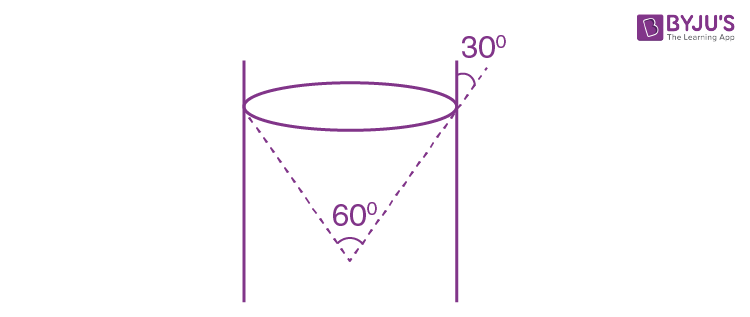$$h=\frac{2Tcos\theta }{\rho gr}\left \{ \theta =30^{0} \right \}$$

$$h=\frac{2\times 0.05\times \times \frac{\sqrt{3}}{2} }{667\times 10\times 0.15\times 10^{-3}}$$

=0.087 m

19. The height ‘h’ at which the weight of a body will be the same as that at the same depth ‘h’ from the surface of the earth is (Radius of the earth is R and effect of the rotation of the earth is neglected):

1. 1)
$$\frac{\sqrt{3}R-R}{2}$$
2. 2)
$$\frac{\sqrt{5}}{2}R-R$$
3. 3)
$$\frac{\sqrt{5}R-R}{2}$$
4. 4)
$$\frac{R}{2}$$

Solution:

$$\frac{g_{0}}{(1+\frac{h}{R})^{2}}=g_{0}\left [ 1-\frac{h}{R} \right ]$$

$$\frac{R^{2}}{(R+h)^{2}}=\left [\frac{R-h}{R} \right ]$$

R3 = (R – h) (R + h)2

R3 = (R –h)(R2+2h+h2)

on solving we get,

$$h=\frac{\sqrt{5}R-R}{2}$$

20. The figure shows a region of length ‘l’ with a uniform magnetic field of 0.3 T in it and a proton entering the region with velocity 4 ×105 ms–1 making an angle 60° with the field. If the proton completes 10 revolution by the time it cross the region shown, ‘l’ is close to (mass of proton = 1.67 × 10–27 kg, charge of the proton = 1.6 × 10–19 C)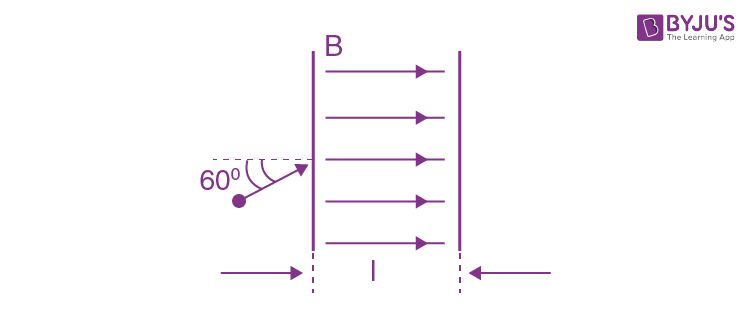1. 1) 0.11 m
2. 2) 0.22 m
3. 3) 0.44 m
4. 4) 0.88 m

Solution:

l = 10 × pitch

= 10 × vcos 60° ×(2π m/qB)

= 10 × v ×(1/2) ×(2πm/qB)

$$l=\frac{10v\pi m}{qB}=\frac{10\times 4\times 10^{5}\times 3.14\times 1.67\times 10^{-27}}{1.6\times 10^{-19}\times 0.3}\simeq 0.44 m\simeq$$

21. A light ray enters a solid glass sphere of refractive index μ= √3 at an angle of incidence 60°. The ray is both reflected and refracted at the farther surface of the sphere. The angle (in degrees) between the reflected and refracted rays at this surface is ________.

Solution: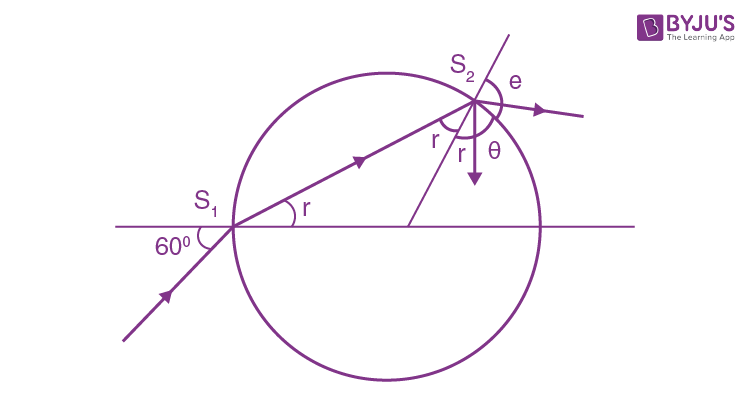At S1

1 x sin 600=√3 sin r

r=300

r = 30°

r1 = 30° {from geometry}

As S2√3 sinr1 = 1sin e

e = 60°

Now, r1 + θ + e = 180°

Θ = 900

22. An ideal cell of emf 10 V is connected in circuit shown in figure. Each resistance is 2Ω. The potential difference (in V) across the capacitor when it is fully charged is _______.

Solution:

1.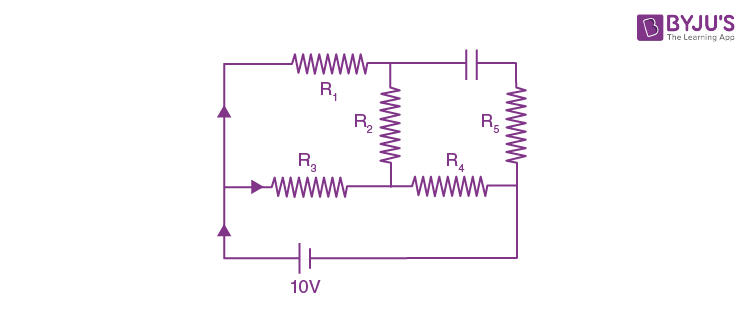Solution: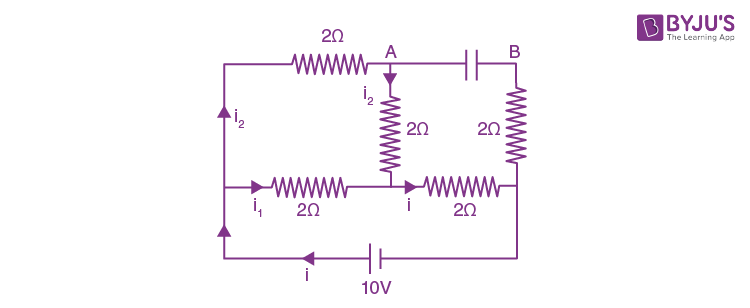$$i=\frac{10}{\frac{4}{3}+2}=\frac{10\times 3}{10}=3Amp$$

i1=2 Amp

i2=1 Amp

VAB = 1 × 2 + 3 × 2 = 8V

23. A square shaped hole of side l =a/2 is carved out at a distance d = a/2 from the centre ‘O’ of a uniform circular disk of radius a. If the distance of the centre of mass of the remaining portion from O is– a/X, value of X (to the nearest integer) is _________.Solution:

$$X_{cm}=\frac{\pi a^{2}\times 0-\frac{a^{2}}{4}\times \frac{a}{2}}{\pi a^{2}-\frac{a^{2}}{4}}$$

$$X_{cm}=\frac{-a}{2(4\pi -1)}=\frac{-a}{8\pi -2}$$

X= (8 π-2) = 8 x 3.14 -2

= 23.12

Nearest Integer = 23

24. A particle of mass m is moving along the x-axis with initial velocity

$$u\hat{i}$$
. It collides elastically with a particle of mass 10 m at rest and then moves with half its initial kinetic energy (see figure). If sin θ1 =√ n sin θ2 then value of n is

Solution:

1.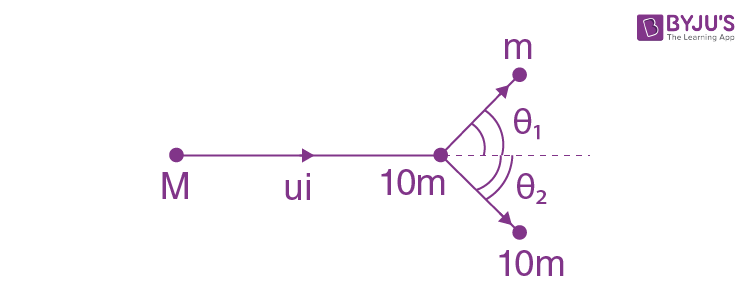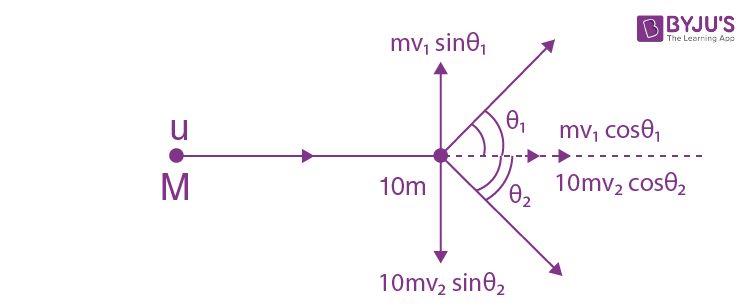$$\frac{1}{2}mv_{1}^{2}=\frac{1}{2}\left ( \frac{1}{2}mu^{2} \right )$$

$$v_{1}^{2}=u^{2}/2$$

$$v_{1}=u/\sqrt{2}$$
--------(i)

Also,

$$\frac{1}{2}mu^{2}=\frac{1}{2}mv_{1}^{2}+\frac{1}{2}\times 10m\times v_{2}^{2}$$

$$\frac{1}{2}\times 10m\times v_{2}^{2}=\frac{1}{2}\times \frac{1}{2}mu^{2}$$

$$v_{2}^{2}=\frac{u^{2}}{20}$$

$$v_{2}=\frac{u}{\sqrt{20}}$$

Now, mv1sin θ1= 10 mv2sin θ2

(u/√2)sin θ1= 10 x (u/√20)sin θ2

sin θ1=(10/√10)sin θ2

sin θ1=√10sin θ2

n= 10

25. A wire of density 9 × 10–3 kg cm–3 is stretched between two clamps 1 m apart. The resulting strain in the wire is 4.9 × 10–4. The lowest frequency of the transverse vibrations in the wire is (Young’s modulus of wire Y = 9 ×1010 Nm–2), (to the nearest integer), _______.

Solution:

$$f=\frac{1}{2l}\sqrt{\frac{T}{\mu }}=\frac{1}{2l}\sqrt{\frac{T}{\rho A}}=\frac{1}{2l}\sqrt{\frac{Y\Delta l}{\rho l}}$$

$$f=\frac{1}{2\times 1}\sqrt{\frac{9\times 10^{10}\times 4.9\times 10^{-4}}{9000\times 1}}=35 Hz$$

### JEE Main 2020 Physics Paper With Solutions Shift 2 September 2